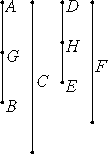# Proposition 6

If a number is parts of a number, and another is the same parts of another, then the sum is also the same parts of the sum that the one is of the one.

Let the number AB be parts of the number C, and another number DE be the same parts of another number F that AB is of C.

I say that the sum of AB and DE is also the same parts of the sum of C and F that AB is of C.Since there are as many parts of DE in F as there are parts AB is of C, therefore there are as many parts of F in DE as there are parts of C in AB.

Divide AB into the parts of C, namely AG and GB, and divide DE into the parts of F, namely DH, and HE. Then the multitude of AG and GB equals the multitude of DH and HE.

VII.5

And since DH is the same part of F that AG is of C, therefore the sum of AG and DH is the same part of the sum of C and F that AG is of C. For the same reason, the sum of GB and HE is the same parts of the sum of C and F that GB is of C.

Therefore the sum of AB and DE is the same parts of the sum of C and F that AB is of C.

Therefore, if a number is parts of a number, and another is the same parts of another, then the sum is also the same parts of the sum that the one is of the one.

Q.E.D.

## Guide

This proposition says multiplication by fractions distributes over addition. Algebraically, if a = (m/n)b and d = (m/n)e then a + d = (m/n)(b + e). As an equation, this says

(m/n)b + (m/n)e = (m/n)(b + e).

#### Use of this proposition

This proposition is used in a few of the propositions coming up starting with VII.9.